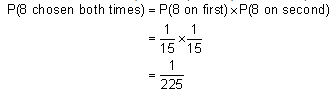## What is the probability that they will both come to a party, Mathematics

Assignment Help:

Q. Suppose the probability of David coming to a party is 75% and the probability of Jason coming to a party is 85%. What is the probability that they will both come to a party, assuming the events are independent?

Solution:

Since the events are independent, we know that
P(David and Jason) = P(David) x P(Jason) = 0.75 x 0.85 = 0.64.
There is a 64% probability that both David and Jason will come to a party.

Example: The 15 labeled pool balls are put into a large bin. One ball is selected at random and put back into the box, and then another ball is chosen at random. What is the probability that the black 8 ball is selected both times?

Solution: Since the first ball is replaced before the second is chosen, both picks are independent of each other. Thus P(8 on first) and P(8 on second) are independent.#### Fractions, what is equizilent to 2/5

what is equizilent to 2/5

#### Area related to circles, railway tunnel of radius 3.5 m and angle aob =90 f...

railway tunnel of radius 3.5 m and angle aob =90 find height of the tunnel

#### Dot product - vector, Dot Product- Vector The other topic for discu...

Dot Product- Vector The other topic for discussion is that of the dot product.  Let us jump right into the definition of dot product. There is given that the two vectors a

#### Number theory, modular arithematic

modular arithematic

#### Sketch the feasible region, Sketch the feasible region for the following se...

Sketch the feasible region for the following set of constraints: 3y - 2x  ≥ 0 y + 8x  ≤  53 y - 2x  ≤  2 x  ≥ 3. Then find the maximum and minimum values of the objective

#### Algebraic expressions word problems, Juan is g years old and Eva is 2 years...

Juan is g years old and Eva is 2 years younger than Juan. a.Find the sum of their ages in terms of g. b.Find the sum of their ages in g years'' time,in terms of g.

#### Dy/dx, how do you differentiate sinx/ex?

how do you differentiate sinx/ex?

(x+y+1)dy/dx=1

#### Rate of change interpretation of derivative, Rate of Change : The first in...

Rate of Change : The first interpretation of derivative is rate of change.  It was not the primary problem which we looked at in the limit chapter, however it is the most signific

#### Linear approximations, Linear Approximations In this section we will l...

Linear Approximations In this section we will look at an application not of derivatives but of the tangent line to a function. Certainly, to get the tangent line we do have to